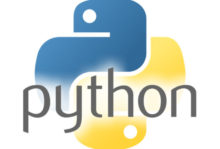# Python “to be define”Example 1 of how to simplify a code :

```vars = [1,2,3]
result = [num * 2]

for var in vars:
result.append(num * 2)

It can be simplify by :

result = [num * 2 for var in vars]

Example 2 of how to simplify a code :

vars= ["patrick","bob","erick","sonia","bruce","laurent",]
result = []

for each_element in vars:
if vars.startwith("s"):
result.append(each_element)
print(result)

It can be simplify by :

result = [vars for each_element in vars if vars.startwith("s")]

Example 3 of how to simplify a code :

def func1():
name = "patrick"
if name == "patrick":
return name

It can be simplify by :

return name, name == "patrick"

```

Example 4 of how to simplify a code :

```def func1(x):
return x * 2

list1 = [1, 2, 3, 4]```
```for each_element in list1:
result = func1(each_element)
print(result)```
```It can be simplify by :

```
```result2 = [func1(each_element) for each_element in list1]
print(result2)

or even :

result3 = map(list[func1, list1))

or you could make a lambda instead of that function :

result3 = map(list(lambda x:x * 2, list1))

Example 5 of how to simplify a code :```
```users = {"bob": "password","mary": "botcat", "rich": "marchs", "sunny": "wheel"}

for item in users.items():
print(item)

It can be simplify by :

username_mapping = {value: value for value in users}

` `